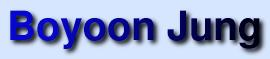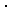Boyoon Jung's Library Manual

# bj::Joystick Class Reference [Sensor Device Driver]

A device driver for joystick devices. More...

`#include <joystick.h>`

List of all members.

## Public Member Functions

Joystick (char *device="/dev/js0")
A constructor.
~Joystick (void)
A destructor.
bool open (char *device)
Open a joystick device.
bool close (void)
Close the device file.
float axis (int index)
Retrieve the scaled value of the specific axis.
float x (void)
Retrieve the scaled value of the X-axis whose index is 0.
float y (void)
Retrieve the scaled value of the Y-Axis whose index is 1.
float z (void)
Retrieve the scaled value of the Z-Axis whose index is 2.
float x2 (void)
Retrieve the scaled value of the second X-Axis whose index is 2.
float y2 (void)
Retrieve the scaled value of the second Y-Axis whose index is 3.
int button (int index)
Check if the indexed button is pressed.
int rawAxis (int index)
Retrieve the raw value (-32767 ~ 32767) of a specific axis.
int rawX (void)
Retrieve the raw value (-32767 ~ 32767) of the X-axis whose index is 0.
int rawY (void)
Retrieve the raw value (-32767 ~ 32767) of the Y-axis whose index is 1.
int rawZ (void)
Retrieve the raw value (-32767 ~ 32767) of the Z-axis whose index is 2.
int rawX2 (void)
Retrieve the raw value (-32767 ~ 32767) of the second X-axis whose index is 2.
int rawY2 (void)
Retrieve the raw value (-32767 ~ 32767) of the second Y-axis whose index is 3.
void range (int axis, float &mn, float &mx)
Retrieve the range setting of a specific axis.
void xRange (float &mn, float &mx)
Retrieve the range setting of the X-axis whose index is 0.
void yRange (float &mn, float &mx)
Retrieve the range setting of the Y-axis whose index is 1.
void zRange (float &mn, float &mx)
Retrieve the range setting of the Z-axis whose index is 2.
void x2Range (float &mn, float &mx)
Retrieve the range setting of the second X-axis whose index is 2.
void y2Range (float &mn, float &mx)
Retrieve the range setting of the second Y-axis whose index is 3.
float minRange (int axis=0)
Retrieve the minimum range setting of a specific axis.
float maxRange (int axis=0)
Retrieve the maximum range setting of a specific axis.
float minXRange (void)
Retrieve the minimum range setting of the X-axis whose index is 0.
float maxXRange (void)
Retrieve the maximum range setting of the X-axis whose index is 0.
float minYRange (void)
Retrieve the minimum range setting of the Y-axis whose index is 1.
float maxYRange (void)
Retrieve the maximum range setting of the Y-axis whose index is 1.
float minZRange (void)
Retrieve the minimum range setting of the Z-axis whose index is 2.
float maxZRange (void)
Retrieve the maximum range setting of the Z-axis whose index is 2.
float minX2Range (void)
Retrieve the minimum range setting of the second X-axis whose index is 2.
float maxX2Range (void)
Retrieve the maximum range setting of the second X-axis whose index is 2.
float minY2Range (void)
Retrieve the minimum range setting of the second Y-axis whose index is 3.
float maxY2Range (void)
Retrieve the maximum range setting of the second Y-axis whose index is 3.
void setRange (float minRange, float maxRange)
Set the same range for all axes.
void setRange (int axis, float minRange, float maxRange)
Set the range of a specific axis.
void setXRange (float minRange, float maxRange)
set the range of the X-axis whose index is 0.
void setYRange (float minRange, float maxRange)
set the range of the Y-axis whose index is 1.
void setZRange (float minRange, float maxRange)
set the range of the Z-axis whose index is 2.
void setX2Range (float minRange, float maxRange)
set the range of the second X-axis whose index is 2.
void setY2Range (float minRange, float maxRange)
set the range of the second Y-axis whose index is 3.
int axes (void)
Return the number of axes available.
int buttons (void)
Return the number of buttons available.
int version (void)
Return the joystick driver version.
void version (char vstr[])
Return the joystick driver version in a string form.
const char * driver (void)
Return the joystick driver name.
void driver (char *buffer)
Return the joystick driver name.

## Detailed Description

A device driver for joystick devices.

Joystick class provides a simpler interface to joystick devices.

## Constructor & Destructor Documentation

 bj::Joystick::Joystick ( char * device = `"/dev/js0"` )
 A constructor.

 bj::Joystick::~Joystick ( void )
 A destructor.

## Member Function Documentation

 int bj::Joystick::axes ( void ) ` [inline]`
 Return the number of axes available.

 float bj::Joystick::axis ( int index ) ` [inline]`

Retrieve the scaled value of the specific axis.

Parameters:
 index Axis index.

 int bj::Joystick::button ( int index ) ` [inline]`
 Check if the indexed button is pressed.

 int bj::Joystick::buttons ( void ) ` [inline]`
 Return the number of buttons available.

 bool bj::Joystick::close ( void )
 Close the device file.

 void bj::Joystick::driver ( char * buffer ) ` [inline]`
 Return the joystick driver name.

 const char* bj::Joystick::driver ( void ) ` [inline]`
 Return the joystick driver name.

 float bj::Joystick::maxRange ( int axis = `0` ) ` [inline]`
 Retrieve the maximum range setting of a specific axis.

 float bj::Joystick::maxX2Range ( void ) ` [inline]`
 Retrieve the maximum range setting of the second X-axis whose index is 2.

 float bj::Joystick::maxXRange ( void ) ` [inline]`
 Retrieve the maximum range setting of the X-axis whose index is 0.

 float bj::Joystick::maxY2Range ( void ) ` [inline]`
 Retrieve the maximum range setting of the second Y-axis whose index is 3.

 float bj::Joystick::maxYRange ( void ) ` [inline]`
 Retrieve the maximum range setting of the Y-axis whose index is 1.

 float bj::Joystick::maxZRange ( void ) ` [inline]`
 Retrieve the maximum range setting of the Z-axis whose index is 2.

 float bj::Joystick::minRange ( int axis = `0` ) ` [inline]`
 Retrieve the minimum range setting of a specific axis.

 float bj::Joystick::minX2Range ( void ) ` [inline]`
 Retrieve the minimum range setting of the second X-axis whose index is 2.

 float bj::Joystick::minXRange ( void ) ` [inline]`
 Retrieve the minimum range setting of the X-axis whose index is 0.

 float bj::Joystick::minY2Range ( void ) ` [inline]`
 Retrieve the minimum range setting of the second Y-axis whose index is 3.

 float bj::Joystick::minYRange ( void ) ` [inline]`
 Retrieve the minimum range setting of the Y-axis whose index is 1.

 float bj::Joystick::minZRange ( void ) ` [inline]`
 Retrieve the minimum range setting of the Z-axis whose index is 2.

 bool bj::Joystick::open ( char * device )
 Open a joystick device.

 void bj::Joystick::range ( int axis, float & mn, float & mx ) ` [inline]`
 Retrieve the range setting of a specific axis.

 int bj::Joystick::rawAxis ( int index ) ` [inline]`
 Retrieve the raw value (-32767 ~ 32767) of a specific axis.

 int bj::Joystick::rawX ( void ) ` [inline]`
 Retrieve the raw value (-32767 ~ 32767) of the X-axis whose index is 0.

 int bj::Joystick::rawX2 ( void ) ` [inline]`
 Retrieve the raw value (-32767 ~ 32767) of the second X-axis whose index is 2.

 int bj::Joystick::rawY ( void ) ` [inline]`
 Retrieve the raw value (-32767 ~ 32767) of the Y-axis whose index is 1.

 int bj::Joystick::rawY2 ( void ) ` [inline]`
 Retrieve the raw value (-32767 ~ 32767) of the second Y-axis whose index is 3.

 int bj::Joystick::rawZ ( void ) ` [inline]`
 Retrieve the raw value (-32767 ~ 32767) of the Z-axis whose index is 2.

 void bj::Joystick::setRange ( int axis, float minRange, float maxRange ) ` [inline]`
 Set the range of a specific axis.

 void bj::Joystick::setRange ( float minRange, float maxRange ) ` [inline]`
 Set the same range for all axes.

 void bj::Joystick::setX2Range ( float minRange, float maxRange ) ` [inline]`
 set the range of the second X-axis whose index is 2.

 void bj::Joystick::setXRange ( float minRange, float maxRange ) ` [inline]`
 set the range of the X-axis whose index is 0.

 void bj::Joystick::setY2Range ( float minRange, float maxRange ) ` [inline]`
 set the range of the second Y-axis whose index is 3.

 void bj::Joystick::setYRange ( float minRange, float maxRange ) ` [inline]`
 set the range of the Y-axis whose index is 1.

 void bj::Joystick::setZRange ( float minRange, float maxRange ) ` [inline]`
 set the range of the Z-axis whose index is 2.

 void bj::Joystick::version ( char vstr[] ) ` [inline]`
 Return the joystick driver version in a string form.

 int bj::Joystick::version ( void ) ` [inline]`
 Return the joystick driver version.

 float bj::Joystick::x ( void ) ` [inline]`
 Retrieve the scaled value of the X-axis whose index is 0.

 float bj::Joystick::x2 ( void ) ` [inline]`
 Retrieve the scaled value of the second X-Axis whose index is 2.

 void bj::Joystick::x2Range ( float & mn, float & mx ) ` [inline]`
 Retrieve the range setting of the second X-axis whose index is 2.

 void bj::Joystick::xRange ( float & mn, float & mx ) ` [inline]`
 Retrieve the range setting of the X-axis whose index is 0.

 float bj::Joystick::y ( void ) ` [inline]`
 Retrieve the scaled value of the Y-Axis whose index is 1.

 float bj::Joystick::y2 ( void ) ` [inline]`
 Retrieve the scaled value of the second Y-Axis whose index is 3.

 void bj::Joystick::y2Range ( float & mn, float & mx ) ` [inline]`
 Retrieve the range setting of the second Y-axis whose index is 3.

 void bj::Joystick::yRange ( float & mn, float & mx ) ` [inline]`
 Retrieve the range setting of the Y-axis whose index is 1.

 float bj::Joystick::z ( void ) ` [inline]`
 Retrieve the scaled value of the Z-Axis whose index is 2.

 void bj::Joystick::zRange ( float & mn, float & mx ) ` [inline]`
 Retrieve the range setting of the Z-axis whose index is 2.

The documentation for this class was generated from the following file:
ResearchProjectsPublications

ToolsBjLib ManualPlayer/StagePyPlayerMicroStrain IMUGPS/DGPSParticle Filter

PersonalWelcomeCurriculum VitaeResumeContact

Outside LinksUSCUSC CS DeptCRESRobotics LabRESL